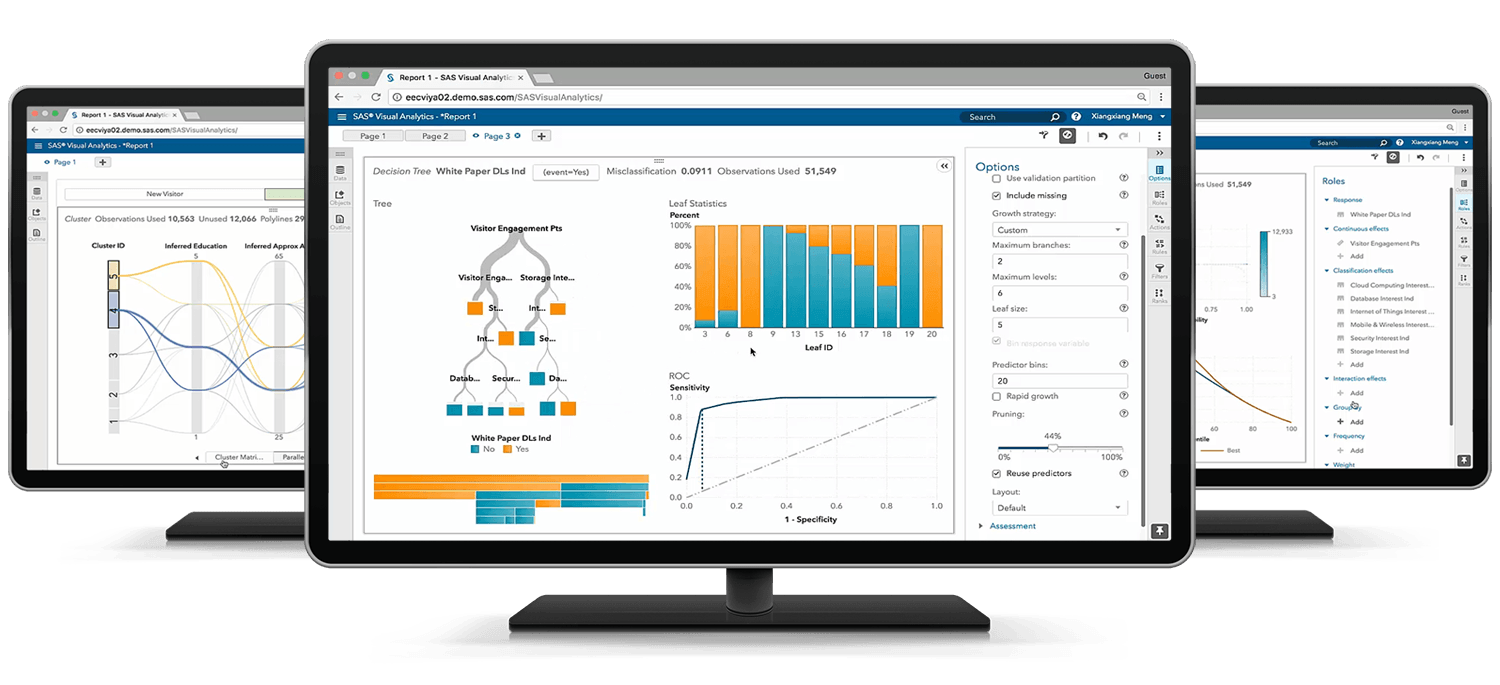# SAS Interactive Model Building and Exploration Using SAS Visual Statistics 7.4

## Exam Content Guide

Below we provide a list of the objectives that will be tested on the exam.## SAS visual statistics cross-functional tasks - 18%

• Prepare data using SAS Visual Analytics
• Filter data used for a model
• Use interactive group-by

## Building and assessing segmentation models - 32%

• Perform unsupervised segmentation using cluster analysis
• Analyze cluster results
• Perform supervised segmentation using decision trees
• Asses decision tree results

## Building and assessing regression-type models - 40%

• Explain linear models
• Perform linear regression modeling
• Perform generalized linear regression modeling
• Perform logistic regression modeling
• Assess model results

## Model comparison and scoring - 10%

• Compare Models
• Score Models